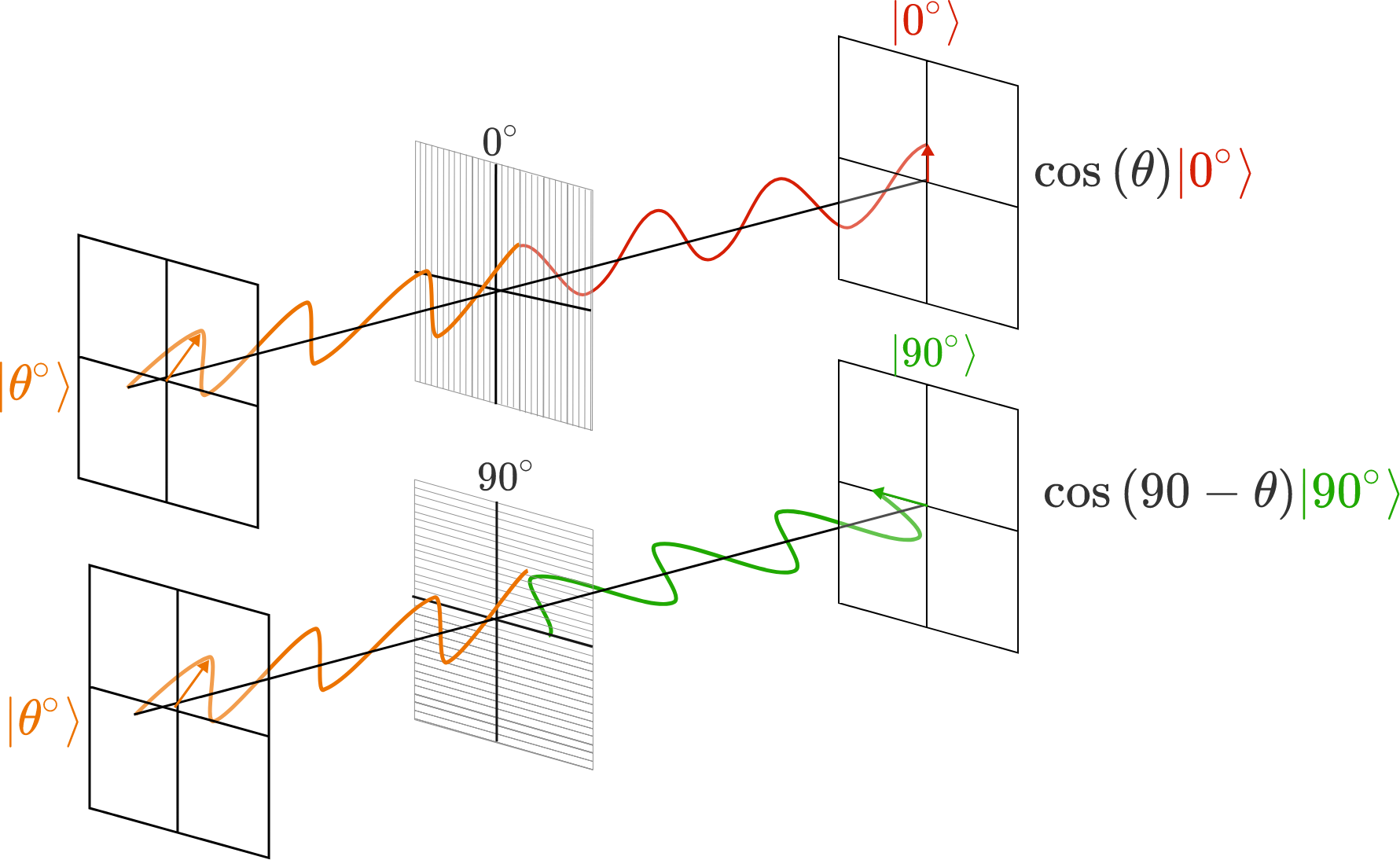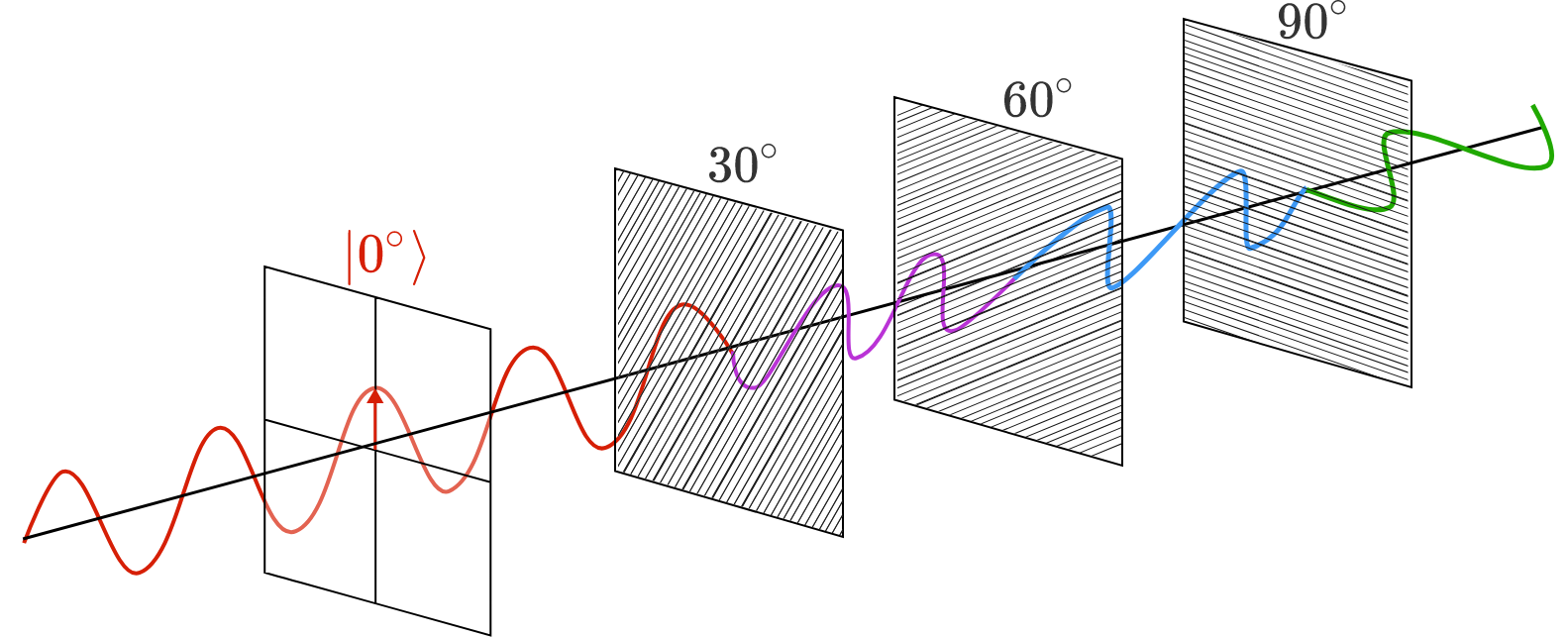# Quantum Computing 1.3 -- Polarizers

A two-state quantum system is called a qubit. A qubit can be realized by a photon which has two different polarization states $\ket{0^{\circ}}$ and $\ket{90^{\circ}}$ which correspond to polarization along the vertical and horizontal axes, respectively. The ability to precisely and reliably manipulate qubits is key to the advent of large-scale quantum computing

A linearly polarized photon aligned with angle $\theta$ can be split into its $\ket{0^{\circ}}$ and $\ket{90^{\circ}}$ components with amplitudes corresponding to the projection of the wave on the measurement axis. These states are orthogonal, so a pure $\ket{0^{\circ}}$ photon has no $\ket{90^{\circ}}$ component, and vice versa: $\ket{\theta ^ \circ} = \cos(\theta) \ket{0^{\circ}} + \cos(90 ^ \circ -\theta) \ket{90^{\circ}}, \quad \theta \in [0^\circ,360^\circ].$One way to manipulate a photon qubit is with polarization filters. Each filter measures and absorbs all photons in the $\ket{\alpha+90 ^ \circ}$ state, while all others pass through. Consider a series of three linear polarization filters, each rotated by an angle of $\alpha = 30 ^ \circ, 60 ^ \circ,$ and $90 ^ \circ,$ respectively, with respect to the $\ket{0^{\circ}}$ polarization direction.What is the probability $P$ that a $\ket{0^{\circ}}$ photon passes all three polarizing filters and emerges as a $\ket{90^{\circ}}$ photon?

×